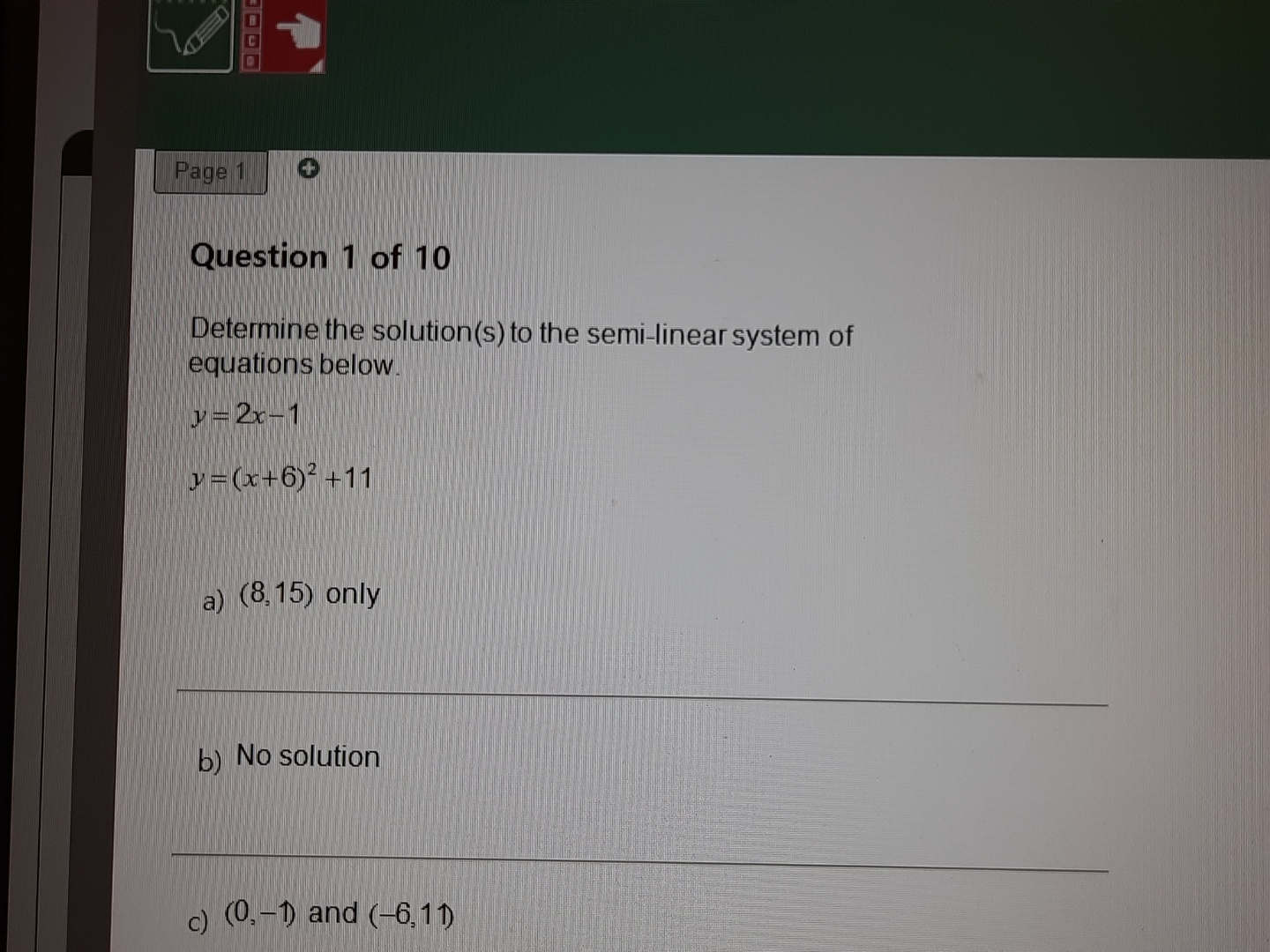## When Helping Means Winning contest

Ask a school-related question in the Help Zone and you could win a \$1000 scholarship!

# Help Zone

### Student QuestionThis is semi linear system and I don't know what to do

Mathematics## Explanations (1)

•Explanation from AlloprofExplanation from Alloprof

This Explanation was submitted by a member of the Alloprof team.

Hello Red Ruby!

$$y = y \Rightarrow 2x - 1 = (x+6)^2 + 11$$
You can then isolate all the equations on one side to have an equation following the format $$0 = ax^2 + bx + c$$. You can then find the value for $$b^2 - 4ac$$ to determine of the system will admit one, two or no solutions.